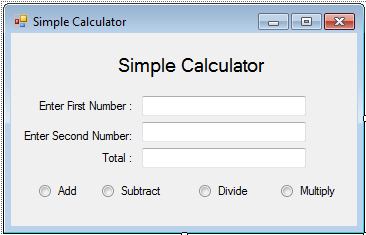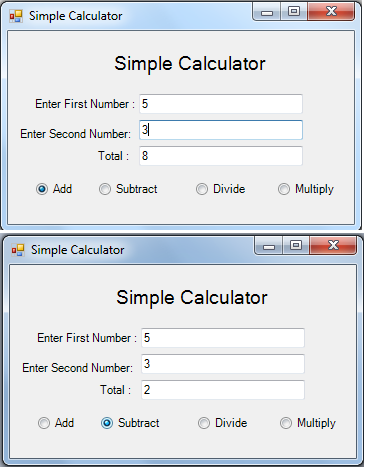# Simple Calculator using a Class in C#

In this C# tutorial, I will teach you how to create a program that will compute sum, difference, quotient, and product as just a simple calculator that uses a class.

Now, let's start this tutorial!

1. Let's start with creating a Windows Form Application in C# for this tutorial by following the following steps in Microsoft Visual Studio: Go to File, click New Project, and choose Windows Application.

2. Next, add one TextBox named TextBox1 for inputting first number, Textbox2 for inputting second number, and Textbox3 for displaying the total.Insert also 4 RadioButton named Radio_Addition for addition operation, Radio_Subtraction for subtraction operation, Radio_Division for division operation and Radio_Multiplication for multiplication operation. You must design your layout like this:3. Create a class named calculate and put this code below.

`using System.Diagnostics;using System;using System.Xml.Linq;using System.Windows.Forms;using System.Collections;using System.Drawing;using System.Data;using System.Collections.Generic;using System.Linq;  namespace MDAS{	public class calculate	{		//declare a private variable as a double		private double _num1;		private double _num2;		private double _total; 		//set a readonly property for the total of the MDAS		public double total		{			get			{				return _total; //return the total			}		}		//create a property for a private variable so that you can access it		public double num1		{			get			{				return _num1; //return the first value			}			set			{				_num1 = value; //set the first value			}		}		public double num2		{			get			{				return _num2;			}			set			{				_num2 = value;			}		} 		//create the sub procedures of the MDAS		public void multiply()		{			//formula of multiplication			_total = num1 * num2;		}		public void divide()		{			//formula of division			_total = num1 / num2;		}		public void add()		{			//formula of addition			_total = num1 + num2;		}		public void subtract()		{			//formula of subtraction			_total = num1 - num2;		}  	} }`

4. Now, go to your Form and put this code below for the calculator operation.

`using System.Diagnostics;using System;using System.Xml.Linq;using System.Windows.Forms;using System.Collections;using System.Drawing;using System.Data;using System.Collections.Generic;using System.Linq;   namespace MDAS{	public partial class Form1	{		public Form1()		{			InitializeComponent();		} 		//create a sub procedure for the events of clicking the radio button that handles all of it.		public void RadioButton_Click(object sender, System.EventArgs e)		{ 			//call a constructor method and return to cal as an instance of a class			calculate cal = new calculate(); 			//declaring the string variable represent as a textbox			string txtnum1 = TextBox1.Text;			string txtnum2 = TextBox2.Text;			//declaring the double variable			double dbl_val1 = default(double);			double dbl_val2 = default(double); 			if (Information.IsNumeric(txtnum1) && Information.IsNumeric(txtnum2)) //check if the textbox has a numeric value			{				//convert the string to double				dbl_val1 = double.Parse(txtnum1);				dbl_val2 = double.Parse(txtnum2); 				//get the value of the converted variable				//to pass it into the variable in the class				cal.num1 = dbl_val1;				cal.num2 = dbl_val2; 				//the condition is, if the radiobutton is clicked,				//the operation of MDAS executes.				if (Radio_Multiplication.Checked)				{					//result:					cal.multiply(); //call a subname in a class for multiplying				}				else if (Radio_Division.Checked)				{					//result:					cal.divide(); //call a subname in a class for dividing				}				else if (Radio_Addition.Checked)				{					//result:					cal.add(); //call a subname in a class for adding				}				else if (Radio_Subtaction.Checked)				{					//result:					cal.subtract(); //call a subname in a class for subtracting				}			}			else			{				//the result is:				//if the textbox is empty or has a string value				TextBox3.Text = "Enter a number";				return ;			}			//put the result of the MDAS to a textbox.			TextBox3.Text = cal.total.ToString();		}  	} }`

Output:For more inquiries and need programmer for your thesis systems in any kind of programming languages, just contact my number below.

Best Regards,

Engr. Lyndon R. Bermoy
IT Instructor/System Developer/Android Developer
Mobile: 09079373999
Telephone: 826-9296
E-mail:[email protected]

Subscribe at my YouTube Channel at: Ser Bermz

Visit my website at: www.campcodes.com

### Tags

Note: Due to the size or complexity of this submission, the author has submitted it as a .zip file to shorten your download time. After downloading it, you will need a program like Winzip to decompress it.

Virus note: All files are scanned once-a-day by SourceCodester.com for viruses, but new viruses come out every day, so no prevention program can catch 100% of them.